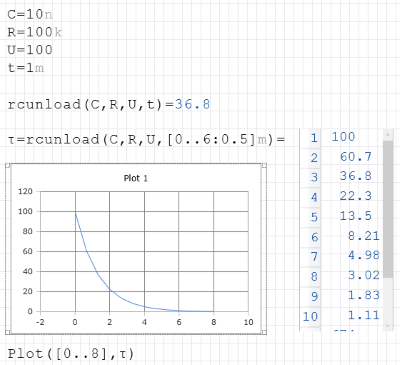Calculats the discharge voltage at a capacitor in a RC circuit

## Calculate discharge voltage

The RCUnload function calculates the discharge voltage on a capacitor of a RC circuid (low pass) at the specified time. The parameters for R and C must be real numbers. Real numbers or lists can be passed for U and t.

### Syntax

RCUnload (R, C, U, List t)

RCUnload (R, C, List U, t)

RCUnload (R, C, List U, List t)

### Legend

 R Resistance in ohms C Capacitor in Farad U Initial voltage in volts t Time in seconds

### Example